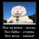צפיות ‎1655‎
Lines

Blue line = SMA = simple moving average
Lime line = SMA percentage selected by the user
Red line = SMA - percentage selected by user

Stop orders

The strategy uses market stop orders. For a backtest, you need to set a fee of the order maker.

Strategy

Reversible system.
If a price is greater than a lime line, open a long position (and close a short position)
If a price is less than a red line, open a short position (and close a long position)
הערות שחרור: + stops

## תגובותДобавил в код выбор таймфрейма!
//Noro
//2019

//@version=4
strategy(title = "Robot WhiteBox StopMA", shorttitle = "Robot WhiteBox StopMA", overlay = true, default_qty_type = strategy.percent_of_equity, default_qty_value = 100, pyramiding = 0)

//Settings
needlong = input(true, defval = true, title = "Long")
needshort = input(false, defval = false, title = "Short")
len = input(50)
src = input(ohlc4)
elpercent = input(5.0, minval = 0, maxval = 100, title = "Shift long, %")
espercent = input(-5.0, minval = -100, maxval = 0, title = "Shift short, %")
showlines = input(true, defval = true, title = "Show lines")
showbg = input(true, defval = true, title = "Show background")

lotsize = input(100, defval = 100, minval = 1, maxval = 10000, title = "Lot, %")
fromyear = input(1900, defval = 1900, minval = 1900, maxval = 2100, title = "From Year")
toyear = input(2100, defval = 2100, minval = 1900, maxval = 2100, title = "To Year")
frommonth = input(01, defval = 01, minval = 01, maxval = 12, title = "From Month")
tomonth = input(12, defval = 12, minval = 01, maxval = 12, title = "To Month")
fromday = input(01, defval = 01, minval = 01, maxval = 31, title = "From day")
today = input(31, defval = 31, minval = 01, maxval = 31, title = "To day")

//Levels
ma = sma(src, len)
el = ma + ((ma / 100) * elpercent)
es = ma + ((ma / 100) * espercent)

//Lines
colel = showlines ? color.lime : na
colma = showlines ? color.blue : na
coles = showlines ? color.red : na
plot(el, color = colel, offset = 1)
plot(ma, color = colma, offset = 1)
plot(es, color = coles, offset = 1)

//Background
trend = 0
trend := high > el ? 1 : low < es ? -1 : trend
colbg = showbg == false ? na : trend == 1 ? color.lime : trend == -1 ? color.red : na
bgcolor(colbg, transp = 80)